Next: Superstrings Up: Perturbative String and Superstring Previous: Spectrum of the Bosonic

## Interacting Strings and the Effective Action

Interacting Strings So far we have described the free propagation of a closed (or open) bosonic string. In what follows we consider the interaction of these strings. Here we focus in the closed string case, the open case requires from further definitions. The interaction of strings at the perturbative level is just the extension of the technique of Feynman diagrams for point particles to extended objets. The vacuum-vacuum amplitude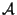is given by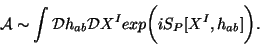(10)

The interacting case requires to sum over all loop diagrams. In the closed string case it means that we have to sum over all compact orientable surfaces. In two dimensions these surfaces are completely characterized by their number of holes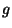(the genus). The relevant topological invariant is the Euler number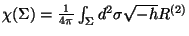, where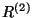is the scalar curvature of the worldsheet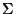. In order to include the interaction of strings, the generalization of the Polyakov action consistent with its symmetries is given by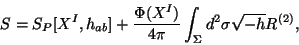(11)

where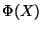is an scalar background field and represents the gravitational coupling constant of the two-dimensional Einstein-Hilbert Lagrangian.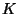in the above equation stands for the geodesic curvature of. If we define the string coupling constant by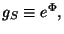then Eq. (10) generalizes to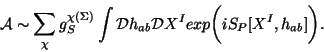(12)

The amplitude defined on-shell correspond to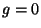and the rest (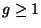) corresponds to-loop corrections. The definition of correlation functions of operators requires of the idea of vertex operators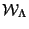. These operators are defined as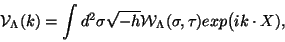(13)

where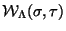(with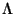being a generic massless field of the bosonic spectra) is a local operator assigned to some specific state of the spectrum of the theory. For instance for the tachyon it is given by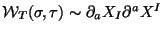. While that for the graviton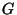with polarization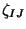it is given by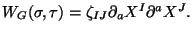Local operators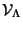are diffeomorphism and conformal invariant and therefore more convenient to define scattering amplitudes. Thus one can define the scattering amplitude of the vertex field operators by their corresponding invariant operators. In perturbation theory the scattering amplitude is given by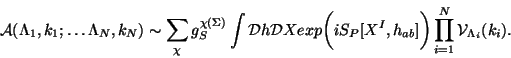(14)

This scattering amplitude is, of course, proportional to the correlation function of the product of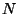invariant operators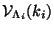as follows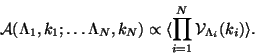(15)

truecm Effective String Actions In order to make contact with the spacetime physics we now describe how the spacetime equations of motion come from conformal invariance conditions for the non-linear sigma model in curved spaces. The immediate generalization of the Polyakov action is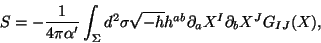(16)

where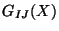is an arbitrary background metric of the curved target space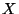. The perturbation of this metric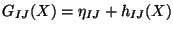in the partition function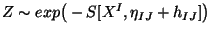, leads to an expansion in powers of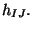This partition function can be easily interpreted as that coming from the interaction of the string with a coherent state of gravitons with invariant operator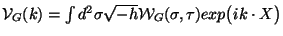with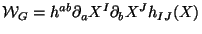. On the other hand, the Polyakov action can be generalized to be consistent with all symmetries and with the massless spectrum of the bosonic closed strings in the form of a non-linear sigma model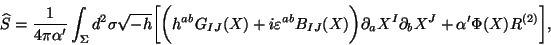(17)

whereis the target space curved metric,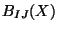is an anti-symmetric field, also called the Kalb-Ramond field, andis the scalar field called the dilaton field. From the viewpoint of the two-dimensional non-linear sigma model these background fields can be regarded as coupling constants and the renormalization group techniques become applied. The computation of the quantum conformal anomaly by using the dimensional regularization method, leads to express the energy-momentum trace as a linear combination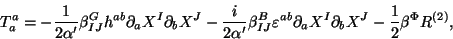(18)

where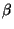are the one-loop beta functions associated with each coupling constant or background field. They are explicitly computed and give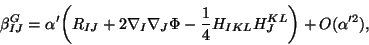(19)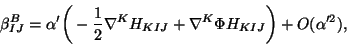(20)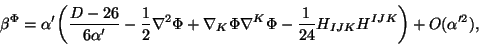(21)

where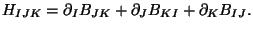Weyl invariance at the quantum level implies the vanishing of the conformal anomaly and therefore the vanishing of each beta function. This leads to three coupled field equations for the background fields. These conditions for these fields can been regarded as equations of motion derivable from the spacetime action in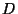dimensions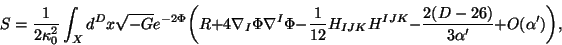(22)

where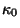is a normalization constant. It is interesting to see that a redefinition of background metric under the transformation indimensions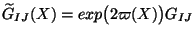with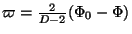leads to the background action in the `Einstein frame'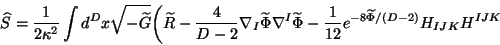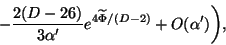(23)

where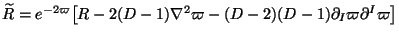and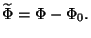The form of this action will of extreme importance later when we describe the strong/weak coupling duality in effective supergravity actions of the different superstring theories types. In the above action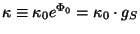is the gravitational coupling constant indimensions, i.e.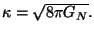A very close procedure can be performed for the open string and compute its effective low energy action. It was done about 15 years ago and for gauge fields with constant field strength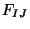it is given by the Dirac-Born-Infeld action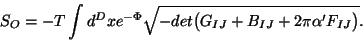(24)

Later we shall say something about some applications of this effective action. truecmNext: Superstrings Up: Perturbative String and Superstring Previous: Spectrum of the Bosonic
root 2001-01-15